# Precalculus : Sum and Difference Identities

## Example Questions

1 3 Next →

### Example Question #21 : Sum And Difference Identities

In the problem below,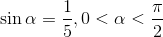and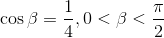.

Find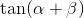.

Possible Answers: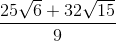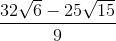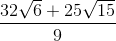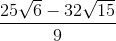Correct answer:Explanation:

Since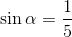and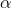is in quadrant I, we can say that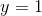and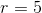and therefore: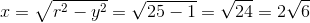So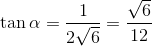.

Since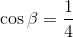and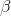is in quadrant I, we can say thatand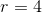and therefore: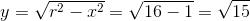.

So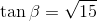Using the tangent sum formula, we see: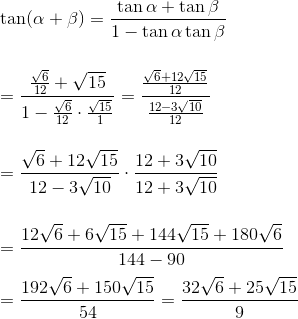### Example Question #22 : Sum And Difference Identities

In the problem below,and.

Find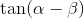.

Possible Answers:Correct answer:Explanation:

Sinceandis in quadrant I, we can say thatandand therefore:So.

Sinceandis in quadrant I, we can say thatandand therefore:So.

Using the tangent sum formula, we see: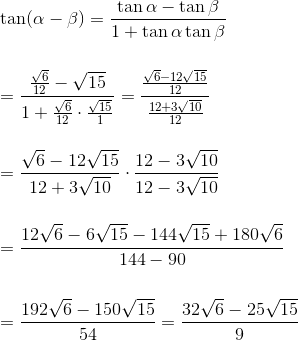### Example Question #23 : Sum And Difference Identities

Given that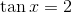and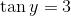, find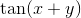.

Possible Answers:Correct answer:Explanation:

Jump straight to the tangent sum formula: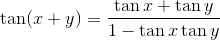From here plug in the given values and simplify.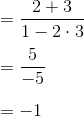### Example Question #21 : Sum And Difference Identities

Which of the following expressions best represents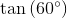?

Possible Answers: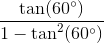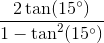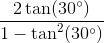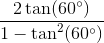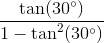Correct answer:Explanation:

Write the identity for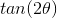.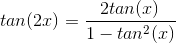Set the value of the angle equal to.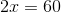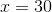Substitute the value ofinto the identity.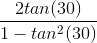### Example Question #25 : Sum And Difference Identities

Find the value of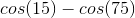.

Possible Answers: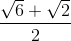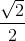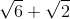Correct answer:Explanation:

To solve, we will need to use both the sum and difference identities for cosine.

Write the formula for these identities.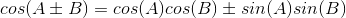To solve for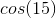and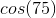, find two special angles whose difference  and sum equals to the angle 15 and 75, respectively.  The two special angles are 45 and 30.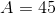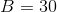Substitute the special angles in the formula.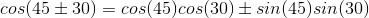Evaluate both conditions.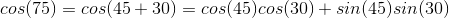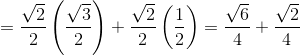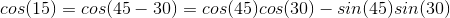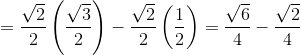Solve for.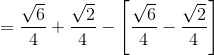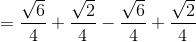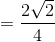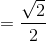1 3 Next →

### All Precalculus Resources